Returns the root nodes in a hierarchical clustering corresponding to a cut defined by a flat cluster assignment vector T. See the fcluster function for more information on the format of T.

For each flat cluster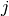of the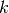flat clusters represented in the n-sized flat cluster assignment vector T, this function finds the lowest cluster nodein the linkage tree Z such that:

• leaf descendents belong only to flat cluster j (i.e. T[p]==j for all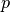in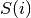whereis the set of leaf ids of leaf nodes descendent with cluster node)
• there does not exist a leaf that is not descendent withthat also belongs to cluster(i.e. T[q]!=j for all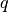not in). If this condition is violated, T is not a valid cluster assignment vector, and an exception will be thrown.
Parameters : Z : ndarray The hierarchical clustering encoded as a matrix. See linkage for more information. T : ndarray The flat cluster assignment vector. A tuple (L, M) with : L : ndarray The leader linkage node id’s stored as a k-element 1D array whereis the number of flat clusters found in T. L[j]=i is the linkage cluster node id that is the leader of flat cluster with id M[j]. If i < n, i corresponds to an original observation, otherwise it corresponds to a non-singleton cluster. For example: if L=2 and M=8, the flat cluster with id 8’s leader is linkage node 2. M : ndarray The leader linkage node id’s stored as a k-element 1D array whereis the number of flat clusters found in T. This allows the set of flat cluster ids to be any arbitrary set ofintegers.

#### Previous topic

scipy.cluster.hierarchy.fclusterdata